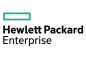New update is available. Click here to update.
Topics

# Count Frequency

Easy0/40
Average time to solve is 15m+18 more companies

## Problem statement

You are given a string 'S' of length 'N', you need to find the frequency of each of the characters from ‘a’ to ‘z’ in the given string.

Example :

``````Given 'S' : abcdg
Then output will be : 1 1 1 1 0 0 1 0 0 0 0 0 0 0 0 0 0 0 0 0 0 0 0 0 0 0
``````
Detailed explanation ( Input/output format, Notes, Images )
Constraints :
``````1 <= T <= 10^2
1 <= Length of the given string <= 10^4
It is guaranteed that all the characters in the string are lower case english alphabets.

Time Limit : 1 sec
``````
Sample Input 1 :
``````1
abaaba
``````
Sample Output 1 :
``````4 2 0 0 0 0 0 0 0 0 0 0 0 0 0 0 0 0 0 0 0 0 0 0 0 0
``````
Explanation For Sample Input 1 :
``````The frequency of ‘a’ is 4 and the frequency of ‘b’ is 2 and rest all characters frequency is 0.
``````
Sample Input 2 :
``````2
bbcccaaaa
z
``````
Sample Output 2 :
``````4 2 3 0 0 0 0 0 0 0 0 0 0 0 0 0 0 0 0 0 0 0 0 0 0 0
0 0 0 0 0 0 0 0 0 0 0 0 0 0 0 0 0 0 0 0 0 0 0 0 0 1
``````
Explanation For Sample Input 2 :
``````For the first input, the frequency of ‘a’ is 4, ‘b’ is 2 and ‘c’ is 3.

For the second input, the frequency of ‘z’ is 1.
``````Console